# Cylindrical Tokamak Equilibrium

Consider a low-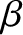, large aspect-ratio, tokamak plasma equilibrium whose magnetic flux-surfaces map out (almost) concentric circles in the poloidal plane. Such an equilibrium can be approximated as a periodic cylinder [4,14]. Let us employ a conventional set of right-handed cylindrical coordinates,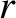,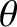,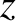. The equilibrium magnetic flux-surfaces lie on surfaces of constant. The system is assumed to be periodic in the(“toroidal”) direction, with periodicity length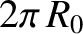, whereis the simulated major radius of the plasma. Let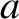be the minor radius of the plasma. The equilibrium magnetic field is written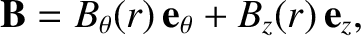(3.1)

where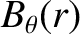is the poloidal magnetic field-strength, and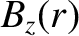the toroidal magnetic field-strength. Here,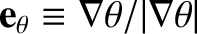and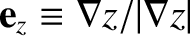. The safety-factor profile takes the form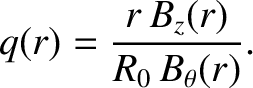(3.2)

[See Equation (1.76).] It is assumed that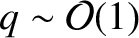. The equilibrium current density is written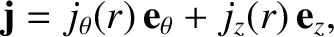(3.3)

where the poloidal and toroidal current densities take the respective forms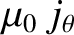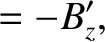(3.4)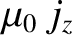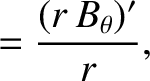(3.5)

anddenotes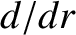. The plasma equilibrium satisfies the force balance criterion [see Equation (2.377)],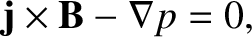(3.6)

where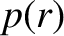is the total plasma pressure. It follows from Equations (3.1) and (3.3)–(3.5) that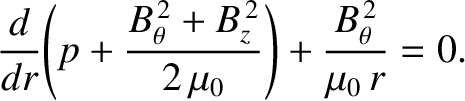(3.7)